﻿ A Possible Answer for the Cosmological Constant ProblemPublications are Open
Access in this journal
Article Versions
Export Article
• Normal Style
• MLA Style
• APA Style
• Chicago Style
Original Article
Open Access Peer-reviewed

A Possible Answer for the Cosmological Constant Problem

Shi-Hao Chen
Frontiers of Astronomy, Astrophysics and Cosmology. 2018, 4(1), 30-31. DOI: 10.12691/faac-4-1-3
Received October 17, 2018; Revised November 20, 2018; Accepted December 04, 2018

Abstract

Based on a model of the universe without singularity, it is proven that the energy density of the vacuum state must be zero or a finite and small positive number. Consequently, the effective cosmological constant is easily determined according to the observations.

1. Introduction

The effective cosmological constant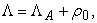here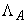and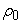are Einstein cosmological constant and the energy density of the ground state of fields (the vacuum state). Λ must be very small or zero. Butis essentially divergent, or very large when high frequency truncation is taken. Consequently, the cosmological constant problem occurs. The problem is not satisfactorily solved. In fact, the problem is hard solved in the conventional theory.

The model of the universe without singularity explains the evolution of the universe 1 based Λ=0, predicts that huge voids have the effects of minus lens. According to this model there is no singularity, but there is the highest temperature in the universe.

We try to solve the cosmological constant problem based on 1.

In section 2 it is proven that the energy density of the ground state must be zero or a finite and small positive number. Section 3 is the conclusion.

2. The Cosmological Constant is Zero or a Small Positive Number

According to 1, there are s-matter and v-matter which are described by the symmetry group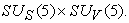Both s-matter and v-matter are symmetric, both masses and energies are positive, but their gravitational masses are opposite from each other. There are two sorts of spontaneously symmetry breaking according to the model which are called s-breaking and v-breaking. When the s-breaking occurs, the gravitational mass density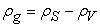, here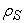and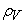are the mass density of s-matter and the mass density of v-matter, respectively. The s-elementary particles get their corresponding proper masses and form s-atoms, s-molecules and s-celestial bodies. The v-elementary are all massless and can only form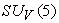color singlets. There is no long range interaction except the gravitational interaction among the v-color singlets. Sach as the interaction by exchanging virtual Higgs particles is short range interaction and very weak so that it can be ignored, because the masses of the Higgs particles in the model are very large. Consequently, the v-color singlets can only diffusely distribute in the universe, cannot be detected and can only be regarded. as dark energy.

The gravitational mass density of the ground state in the nature unit is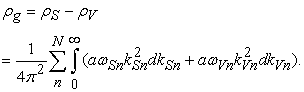(1)

where N is the total number of the degree of freedom of all s- or v- elementary particles, n denotes nth degree of freedom,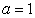for fermions, and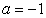for bosons..

Thus, before the symmetry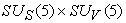is not broken,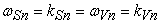(2)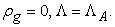(3)

This is because the expectation values of all Higgs fields are zero so that the masses of all elementary particles are zero as well when the symmetryholds, and the total number of the degree of freedom of all s-elementary particles is the same as that of the all v-elementary particles.

Afteris broken to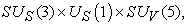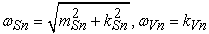(4)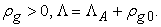(5)

It should be noted that in the case, the total number of the degree of freedom of all s-elementary particles is still the same as that of the all v-elementary particles. For example, after the s-breaking occurs, the degree of freedom of the gauge boson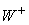is 3 due to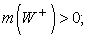Before the s-breaking occurs, the degree of freedom of the gauge boson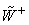corresponding tois 2 due to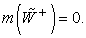But there is a Higgs particle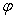which will be eaten byafter s-breaking, i.e.andcombine together to form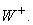The total number of the degree of freedom ofandis the same as that ofIn fact, the total number of the degree of freedom must be conservational, and the total number of the degree of freedom of the s-elementary particles must be the same as that of the v-elementary particle because of the symmetryOn the other hand, it is impossible that a fermion transforms into a boson, and vice versa. Consequently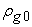must be a finite and small positive number.

If taking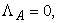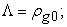If Λ can be determined according to astro observations,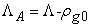must be a small number. It is seen that the model 1 has provided a way to solve cosmological constant problem.

The results are hold as well for the case that the universe is composed of infinite cosmic islands 2.

It is obvious that the above results are true when v-breaking occurs, i.e.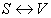.

3. Conclusions

Based on 1, it is proven that the energy densityof the ground state must be zero or a small positive number. Consequently, if taking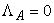,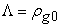; If Λ can be determined according to astronomical observations,must be a small number. It is seen that the model 1 has provided a way to solve cosmological constant problem.

Acknowledgments

The work is supported National Natural Science foundation of China 11075064.

References

  Chen, S.H. “A Cosmological Model without Singularity Based On RW Metric (1)”, International Journal of Astronomy and Astrophysics, 4 (1), 264-293. March 2014. In article View Article  Chen, S.H, Structure of the Universe, to be published in “International Journal of Astronomy and Astrophysics”. In articleThis work is licensed under a Creative Commons Attribution 4.0 International License. To view a copy of this license, visit http://creativecommons.org/licenses/by/4.0/